# Transpose Multiple Columns into One Column

This post will guide you how to transpose multiple columns into a single columns with multiple rows in Excel. How do I put data from multiple columns into one column with Excel formula; How to transpose columns into single column with VBA macro in excel.

Assuming that you have a data list in range B1:D4 contain 3 columns and you want to transpose them into a single column F. just following the below two ways.

## Transpose Multiple Columns into One Column with Formula

You can use the following excel formula to transpose multiple columns that contain a range of data into a single column F:

#1 type the following formula in the formula box of cell F1, then press enter key.

`=INDEX(\$B\$1:\$D\$4,1+INT((ROW(B1)-1)/COLUMNS(\$B\$1:\$D\$4)),MOD(ROW(B1)-1+COLUMNS(\$B\$1:\$D\$4),COLUMNS(\$B\$1:\$D4))+1)`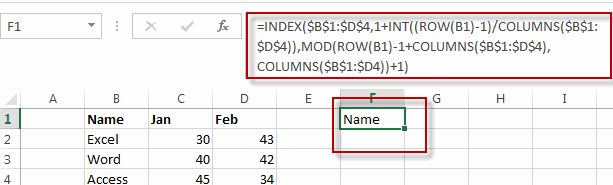#2 select cell F1, then drag the Auto Fill Handler over other cells until all values in range B1:D4 are displayed.

#3 you will see that all the data in range B1:D4 has been transposed into single column F.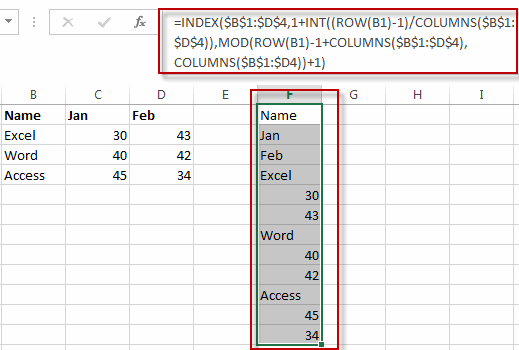## Transpose Multiple Columns into One Column with VBA Macro

You can also write an Excel VBA Macro to transpose the data of range in B1:D4 into single column F quickly. Just do the following steps:

#1 click on “Visual Basic” command under DEVELOPER Tab.#2 then the “Visual Basic Editor” window will appear.

#3 click “Insert” ->”Module” to create a new module.#4 paste the below VBA code into the code window. Then clicking “Save” button.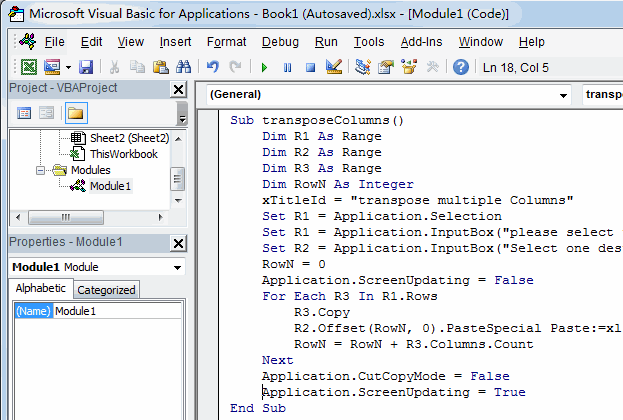```Sub transposeColumns()
Dim R1 As Range
Dim R2 As Range
Dim R3 As Range
Dim RowN As Integer
wTitle = "transpose multiple Columns"
Set R1 = Application.Selection
Set R1 = Application.InputBox("please select the Source data of Ranges:", wTitle, R1.Address, Type:=8)
Set R2 = Application.InputBox("Select one destination single Cell or column:", wTitle, Type:=8)
RowN = 0
Application.ScreenUpdating = False
For Each R3 In R1.Rows
R3.Copy
R2.Offset(RowN, 0).PasteSpecial Paste:=xlPasteAll, Transpose:=True
RowN = RowN + R3.Columns.Count
Next
Application.CutCopyMode = False
Application.ScreenUpdating = True
End Sub```

#5 back to the current worksheet, then run the above excel macro. Click Run button.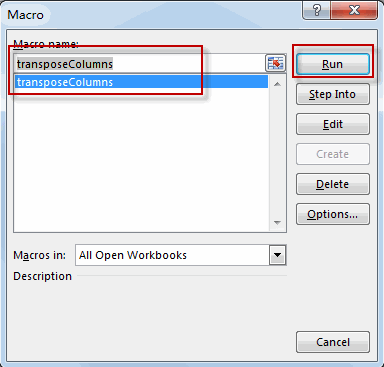#6 select the source data of ranges, such as: B1:D4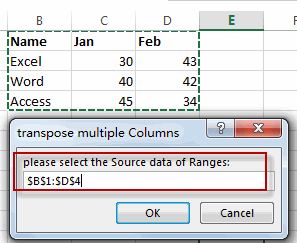#7 select one single cell in the destination Column, such as: F1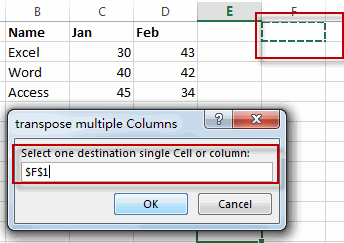#8 let’s see the last result.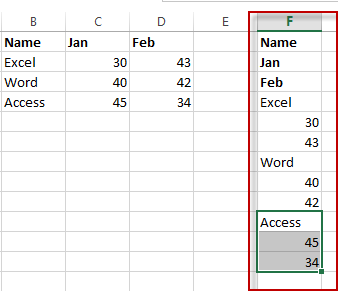### Related Functions

• Excel INDEX function
The Excel INDEX function returns a value from a table based on the index (row number and column number)The INDEX function is a build-in function in Microsoft Excel and it is categorized as a Lookup and Reference Function.The syntax of the INDEX function is as below:= INDEX (array, row_num,[column_num])…
• Excel INT function
The Excel INT function returns the integer portion of a given number. And it will rounds a given number down to the nearest integer.  And the INT function rounds down, so if you provide a negative number, the returned value will become more negative.The syntax of the INT function is as below:= INT (number)…
• Excel ROW function
The Excel ROW function returns the row number of a cell reference.The ROW function is a build-in function in Microsoft Excel and it is categorized as a Lookup and Reference Function.The syntax of the ROW function is as below:= ROW ([reference])….
• Excel Columns function
The Excel COLUMNS function returns the number of columns in an Array or a reference.The syntax of the COLUMNS function is as below:=COLUMNS (array)….
• Excel MOD function
he Excel MOD function returns the remainder of two numbers after division. So you can use the MOD function to get the remainder after a number is divided by a divisor in Excel. The syntax of the MOD function is as below:=MOD (number, divisor)….

Related Posts

Creating a Table with Automatic Row Numbering

When creating an Excel Table, you may use a calculation relying on the ROW function to insert row numbers automatically. The formula in A2, which has been copied down, is as follows: =ROW()-ROW(Table1[#Headers]) Note: the default table name is Table1. ...

Get Address of First Cell in Range

We will learn how to get address of first cell in range in this post. At Instance, we must determine the address of first cell in range to use as the data table's value. For the DATA table, we have ...

Abbreviate Names Or Words in Excel

As an MS Excel user, you might have come across a task where you need to abbreviate different names or words, and there are also possibilities that you might have done this task manually by assuming that there isn't any ...

Filter or Extract with a Partial Match

Assume you have a table consisting of a few cells having few values, and you want to filter out the set of records with the partial match. You might take it easy and would prefer to manually filter out the ...

Extract matching values From Two Lists

Suppose that you are working with two lists containing few values, and you want to extract the matching values from those two lists into another separate list. You might prefer to manually extract the matching values from the two lists, ...

Extract all Partial Matches

Just assume that you have a range of data that contains a text string and you want to extract the few partial matches into another separate range of cells; then you might think that it's not a big deal; because ...

Extract Multiple Match Values into Separate Columns

If you have a few values/items in the excel sheet and you are thinking that with the aid of the “VlOOKUP” function you can look for a specific value, extract it and then put the matching item into the separate ...

Average the Last N Numeric Values in Excel

AVERAGE function is one of the most popular functions in Excel. Apply AVERAGE together with some other functions, we can calculate average simply for some complex situations. In this article, we will introduce you to calculate average of the last ...

How To Sum the Largest N Values in Excel

Sometimes we may want to sum the largest N numbers or top N numbers in a range. In this article, we will show you the method of “SUM the Largest N Numbers” by a simple formula which consist of SUMPRODUCT ...

How to Sum the Smallest N Values in Excel

Sometimes we may want to sum the first smallest N numbers in a range in Excel. In this article, we will show you the method of “SUM the Smallest N Numbers” by a simple formula which consist of SUMPRODUCT and ...

Sidebar